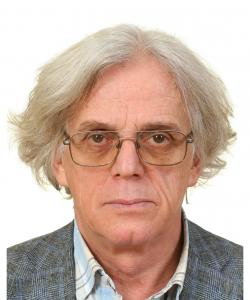Timescales in the Wang-Landau Algorithm
Speaker
Prof. Lev Shchur
Landau Institute for Theoretical Physics, Science Center in Chernogolovka, Russia
Abstract

We review recent advances in the analysis of the Wang-Landau algorithm, which is designed for the direct Monte Carlo estimation of the density of states (DOS). In the case of a discrete energy spectrum, we present an approach based on introducing the transition matrix in the energy space (TMES). The TMES fully describes a random walk in the energy space biased with the Wang-Landau probability. Properties of the TMES can explain some features of the Wang-Landau algorithm, for example, the flatness of the histogram. We show that the Wang-Landau probability with the true DOS generates a Markov process in the energy space and the inverse spectral gap of the TMES can estimate the mixing time of this Markov process. We argue that an efficient implementation of the Wang-Landau algorithm consists of two simulation stages: the original Wang-Landau procedure for the first stage and a 1/t modification for the second stage. The mixing time determines the characteristic time for convergence to the true DOS in the second simulation stage. The parameter of the convergence of the estimated DOS to the true DOS is the difference of the largest TMES eigenvalue from unity. The characteristic time of the first stage is the tunneling time, i.e., the time needed for the system to visit all energy levels.